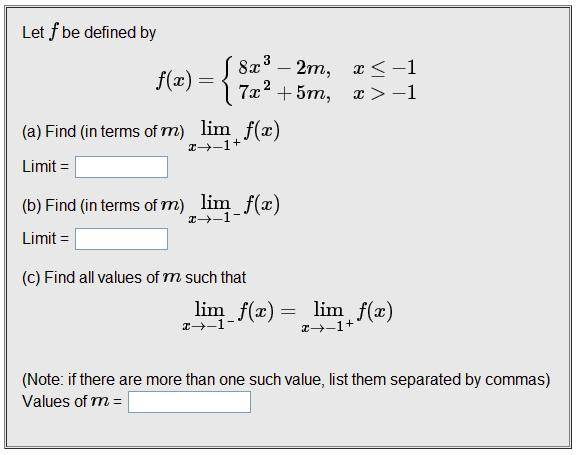# Left & Right Limits Of A Function (Finding Values Of A Variable)

## Homework Statement## Homework Equations

Only ones I know that are relevant are the equations shown in the question (for the left and right limits).

## The Attempt at a Solution

The difficulty that I am having right not is comprehending what is really being asked from the question. From what I understand, then question is asking the "m" values from the left and right limits at the top, and the value for "m" when those limits are the same. However, how would I go about finding the value of "m" if I have no x or y co-ordinates given?

Staff Emeritus
Homework Helper
Gold Member

## Homework Statement## Homework Equations

Only ones I know that are relevant are the equations shown in the question (for the left and right limits).

## The Attempt at a Solution

The difficulty that I am having right not is comprehending what is really being asked from the question. From what I understand, then question is asking the "m" values from the left and right limits at the top, and the value for "m" when those limits are the same. However, how would I go about finding the value of "m" if I have no x or y co-ordinates given?
What is $\displaystyle \lim_{x\to\,1}(8x^3-2m)\ ?$

What is $\displaystyle \lim_{x\to\,1}(8x^3-2m)\ ?$

That is not given (everything above that I posted is the only thing given). Or are you suggesting that I should calculate that to solve this problem. That expression just defines the limit from the left side. I am unsure on how to calculate that.

Staff Emeritus
Homework Helper
Gold Member
That is not given (everything above that I posted is the only thing given). Or are you suggesting that I should calculate that to solve this problem. That expression just defines the limit from the left side. I am unsure on how to calculate that.
What do you mean, it's not given?

I'm asking you to evaluate the limit, $\displaystyle \lim_{x\to\,1}(8x^3-2m)\,,$ if you can. If you can't, I don't how you can do this problem at all.

What do you mean, it's not given?

I'm asking you to evaluate the limit, $\displaystyle \lim_{x\to\,1}(8x^3-2m)\,,$ if you can. If you can't, I don't how you can do this problem at all.

I don't see how I can evaluate that limit... I could isolate "m" though by temporarily assigning "f(x)" as "y". Then I could isolate "m" in terms of "y" => "m(y)".

Mentor
Sammy is not asking you to solve for m (i.e., isolate me) - just evaluate the limit, which by the way should be
$$\lim_{x \to -1^{-}}(8x^3 - 2m)$$

Staff Emeritus
Homework Helper
Gold Member
I don't see how I can evaluate that limit... I could isolate "m" though by temporarily assigning "f(x)" as "y". Then I could isolate "m" in terms of "y" => "m(y)".
$\displaystyle \lim_{x\to\,1}(8x^3-2m) = 8(1)^3-2m=8-2m$

That's all there is to it !